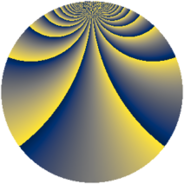# Properties

 Label 1045.2.bjLevel $1045$ Weight $2$ Character orbit 1045.bj Rep. character $\chi_{1045}(109,\cdot)$ Character field $\Q(\zeta_{18})$ Dimension $696$ Sturm bound $240$

# Related objects

## Defining parameters

 Level: $$N$$ $$=$$ $$1045 = 5 \cdot 11 \cdot 19$$ Weight: $$k$$ $$=$$ $$2$$ Character orbit: $$[\chi]$$ $$=$$ 1045.bj (of order $$18$$ and degree $$6$$) Character conductor: $$\operatorname{cond}(\chi)$$ $$=$$ $$1045$$ Character field: $$\Q(\zeta_{18})$$ Sturm bound: $$240$$

## Dimensions

The following table gives the dimensions of various subspaces of $$M_{2}(1045, [\chi])$$.

Total New Old
Modular forms 744 744 0
Cusp forms 696 696 0
Eisenstein series 48 48 0

## Trace form

 $$696 q - 24 q^{4} - 12 q^{5} - 24 q^{9} + O(q^{10})$$ $$696 q - 24 q^{4} - 12 q^{5} - 24 q^{9} - 24 q^{14} + 12 q^{15} - 48 q^{16} - 12 q^{20} - 60 q^{25} - 72 q^{26} - 36 q^{31} - 24 q^{34} + 60 q^{36} + 30 q^{44} - 72 q^{45} - 228 q^{49} + 66 q^{55} + 48 q^{59} + 18 q^{60} + 252 q^{64} - 84 q^{66} - 36 q^{69} + 42 q^{70} - 120 q^{71} - 180 q^{80} - 36 q^{81} + 36 q^{86} + 48 q^{89} + 132 q^{91} - 180 q^{99} + O(q^{100})$$

## Decomposition of $$S_{2}^{\mathrm{new}}(1045, [\chi])$$ into newform subspaces

The newforms in this space have not yet been added to the LMFDB.